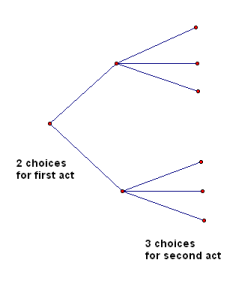﻿ More Probability Concepts

#More Probability Concepts

Need an introduction to probability first?  Basic Probability Concepts

## Properties of Probabilities

Recall that the probability of an event $\,E\,$ is denoted by $\,P(E)\,.$

### Probabilities are Always Numbers Between $\,0\,$ and $\,1\,$

For any event $\,E\,,$ $\,0\le P(E)\le 1\,.$ That is, a probability is always a number between $\,0\,$ and $\,1\,.$

### The Sample Space has Probability $\,1\,$

If $\,S\,$ is the sample space, then $\,P(S)=1\,.$ That is, there's a $\,100\%\,$ chance that something will happen.

### The Meaning of Probabilities $\,0\,$ and $\,1\,$ for Finite Sample Spaces

For a finite sample space (i.e., an experiment with a finite number of outcomes): a probability of $\,1\,$ means the event will definitely occur; a probability of $\,0\,$ means the event will definitely not occur.

Notice that if the experiment is to randomly choose a real number from the interval $\,[0,1]\,,$ then the probability of choosing (say) the number $\,0.5\,$ is zero, and yet this outcome could occur. This is why a finite sample space is needed in the above statement.

### Probability of a Union

For any events $\,A\,$ and $\,B\,$: $$\cssId{s17}{P(A\cup B)} \cssId{s18}{=P(A)+P(B)-P(A\cap B)}$$ If you were just to add the two probabilities, then the intersection gets added twice; this extra probability must be subtracted.

### Probability of the Complement

Recall that $\,\overline{E}\,$ denotes the complement of $\,E\,.$

For any event, either it happens, or it doesn't!

Therefore: $$P(E)+P(\overline{E})=1$$

Equivalently: $$P(\overline{E})=1 -P(E)$$

### Mutually Exclusive Events

Recall that $\,\emptyset \,$ denotes the empty set.

Events $\,A\,$ and $\,B\,$ are said to be mutually exclusive if $\,A\cap B = \emptyset\,.$ That is, two events are mutually exclusive when they have nothing in common (their intersection is empty).

Since $\,P(\emptyset) = 0\,,$ for mutually exclusive events, the above formula is simpler: $$\cssId{s33}{\,P(A\cup B)=P(A)+P(B)}\,$$

## Multiplication Counting PrincipleIf there are $\,F\,$ choices for how to perform a first act, and for each of these $\,F\,$ ways, there are $\,S\,$ choices for how to perform a second act, then there are $\,F\cdot S\,$ ways to perform the acts in succession. (The idea extends to more than $\,2\,$ acts.)

The idea is illustrated by the diagram above. If there are $\,2\,$ piles, with $\,3\,$ in each pile, then the total is $\,2\cdot 3 = 6\,.$

## Example (Pizza Choices)

Suppose that a pizza shop offers $\,3\,$ types of crust, $\,2\,$ different types of cheese, and $\,7\,$ different toppings.

A single-topping pizza consists of a choice of crust, a choice of cheese (if desired), and a choice of topping.

How many different single-topping pizzas are there?

Solution: There are $\,3\,$ choices for the crust, $\,3\,$ choices for cheese (none, first type, second type), and $\,7\,$ choices for the single topping.

By the Multiplication Counting Principle, there are $\,3\cdot 3\cdot 7 = 63\,$ possible single-topping pizzas.